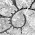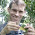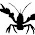## Monday, February 18, 2013

### Visualizing uncertainty in a 'traitgram'

For a variety of a reasons, I've been thinking about clever ways to try and visualize uncertainty in ancestral state estimates. Here is one attempt using an adaptation of the phytools function phenogram. This function plots a projection of the tree into a two dimensional space defined by time since the root (on the x) and phenotype. Note that to run this I had to first add the optional argument add to phenogram, so you will need to download and load the source (phenogram.R) to get this to work.

library(phytools)
source("phenogram.R")
# simulate tree & data
tree<-pbtree(n=20)
plotTree(tree)
x<-fastBM(tree)
# estimate ancestors
A<-fastAnc(tree,x,CI=TRUE)
tree<-paintSubTree(tree,node=length(tree\$tip)+1,"1")
# our transparencies
trans<-as.character(floor(0:50/2))
trans[as.numeric(trans)<10]<-
paste("0", trans[as.numeric(trans)<10],sep="")
# plot
for(i in 0:50){
p<-i/length(trans)
}
One word of caution about this visualization. Since I plot uncertainty using transparent colors, it can be difficult to impossible to extract uncertainty about any specific ancestral node from this plot. However what the plot is good at showing is the probability density of all hypothetical ancestors at any time slice through the tree.

1.Those discontinuities in the probability density at nodes are a function of having multiple (two) lineages and then meeting and becoming one lineage, yes?

Anyway, this is awesome! Made my day.

2.Yes - that's right.

3.This is very cool! also kind of reminds me of Densitree - a way of visualizing gene trees over a species tree

http://www.cs.auckland.ac.nz/~remco/DensiTree/DensiTree.html

4.A slightly more correct way of doing this would be to use the variances instead of the 95% CIs. These are returned by fastAnc in phytools 0.2-20 using fastAnc(...,vars=TRUE). Then, we can just plot the color transparency as a Gaussian (instead of linear) function of the distance from the MLE and the variance on the estimate. I tried this - but with the current approach this too is imperfect because when the variance is low multiple lines of similar transparency overlap creating a darker color and the impression of greater probability density. - Liam

5.So Cool! Definitely going to play with this! Thanks Liam!

6.Hi Liam,

I have been trying to replicate this code as it is here and also with my data and keep getting the following error in both cases:
Error in fastAnc(tree, x, CI = TRUE) : unused argument(s) (CI = TRUE)

Am I doing something wrong?

Thanks!
Ricardo

1.Hi Ricardo.

I'd guess it's because you don't have the latest version of 'phytools'.

My recommendation is that you install the latest minor version (here) from source.

Alternatively, you can install the latest CRAN version (phytools 0.2-20) and then load the source code for phenogram separately.

One side-note. If you are a Mac user, for some reason the phytools 0.2-20 binary was never built by CRAN. If you can't install from source, then you can download the phytools 0.2-20 MacOS X binary from my website.

Let us know if this fixes your problem.

- Liam

Note: due to the very large amount of spam, all comments are now automatically submitted for moderation.